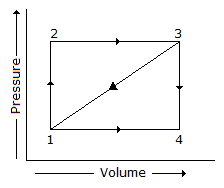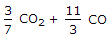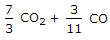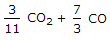# Mechanical Engineering - Thermodynamics

### Exercise :: Thermodynamics - Section 6

1.

The atomic mass of sulphur is

 A. 12 B. 14 C. 16 D. 32

Explanation:

No answer description available for this question. Let us discuss.

2.

A path 1-2-3 is given. A system absorbs 100 kJ as heat and does 60 kJ of work while along the path 1-4-3, it does 20 kJ of work. The heat absorbed during the cycle 1-4-3 isA. -140 kJ B. -80 kJ C. -40 kJ D. +60 kJ

Explanation:

No answer description available for this question. Let us discuss.

3.

The atomic mass of an element is the number of times the atom of that element is heavier than the hydrogen atom.

 A. True B. False

Explanation:

No answer description available for this question. Let us discuss.

4.

The mass of carbon per kg of flue gas is given by

 A.B.C.D.Explanation:

No answer description available for this question. Let us discuss.

5.

A cycle consisting of two isothermal and two isentropic processes, is known as

 A. Carnot cycle B. Stirling cycle C. Ericsson cycle D. Joule cycle